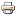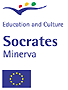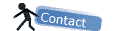# Other astronomy activities## The Solar System as a MathLabThis is a computer-based tool (instead of a hands-on tool like a telescope) to learn and understand some basic mathematics (and physics) behind space travel. The application is splitted into two:

Sailing the Solar System:

Motivated by the recent space experiments to develop Space-Sail Ships as COSMOS-I, this application trains students in geometry, conical functions (ellipses) and iterative processes/converging series.  The application is designed for various levels of expertise:

1st level is the direct use of the tool, e.g.,  training the mathematics contents in a straightforward manner
2nd. level is to use it as a means to teach high level concepts related to geometry (which is the optimum manner to fill a circle with regular poligons), space navigation (what is, and why there are launching windows for space missions) and even numerical method, as the Newton Method, to solve equations.

The Efficient Space Traveller:

Motivated by travelling among the known extra-solar planetary systems, this application trains students in coordinate systems (spherical versus cartessian), vectorial algebra, permutations and the concept of heuristics through well-known "traveller problem" . The application again is designed for various levels of expertise:

1st level is the direct use of the tool, e.g. training the mathematics contents in a straightforward manner

2nd. level is to use it to motivate students to think and apply other heuristics methods.

Theer are three manuals attached to the application to familiarise teachers with the concepts behind the tool (astronomical coordinates, radiation pressure, 2-bodies problem ...) .